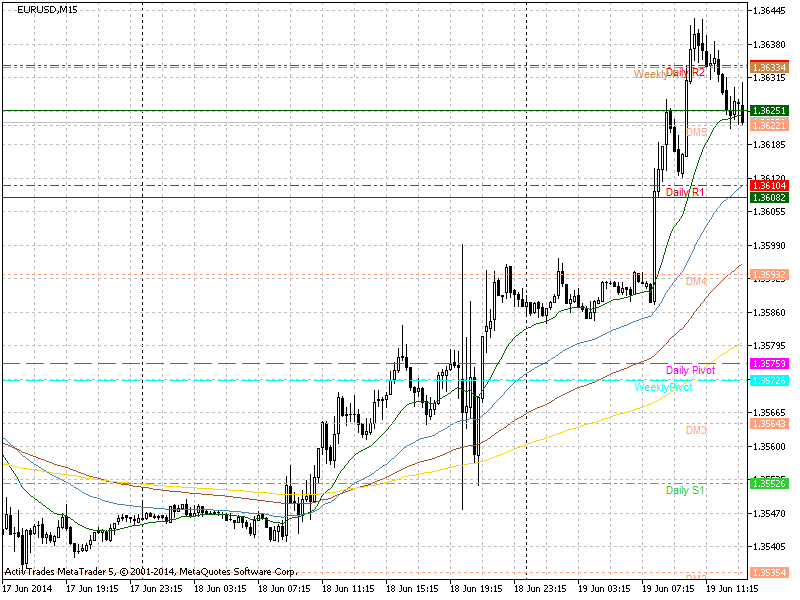# forex software

Create and Test Forex Strategies

forex software

trading:pivot-points

# Pivot Points

Pivot points are significant price levels that are used for determining of support and resistance. The central pivot is calculated by the following formula:

`  PP = (High + Low + Close)/3`

Three additional levels of support (S1, S2, S3) and resistance (R1, R2, R3) are calculated by subtracting or adding price differentials calculated from previous trading ranges of the market. These levels are calculated by the following formulas:

```  R1 = P + (P − L) = 2×P − L
S1 = P − (H − P) = 2×P − H
R2 = P + (H − L)
S2 = P − (H − L)
R3 = H + 2×(P − L) = R1 + (H − L)
S3 = L − 2×(H − P) = S1 − (H − L)

Where:

P - Central pivot
H - High
L - Low
C - Close```

When the price is above the central pivot point, the sentiment is bullish, while when it is below - the sentiment is bearish. The support and resistance levels calculated from the central pivot point can be used as entry or exit points of trades. If you use them as entry points it is better if at the same price level are concentrated other indicators such as swing highs and lows, or moving averages. You can look also for levels of confluence of pivot points calculated on different time frames.

You can calculated the pivot points with online calculators or use custom indicators for MT or other trading and charting platforms.

Pivot points